2018-11-23 19:39:43 shanwenkang 阅读数 1324
• ###### 携手STM32CubeMX玩转STM32

754 人正在学习 去看看 李凯龙

# 二维傅里叶变换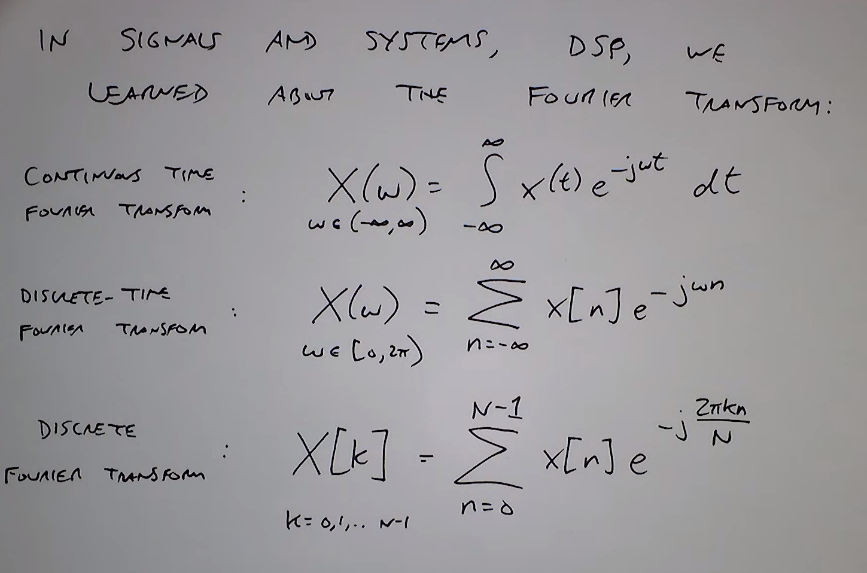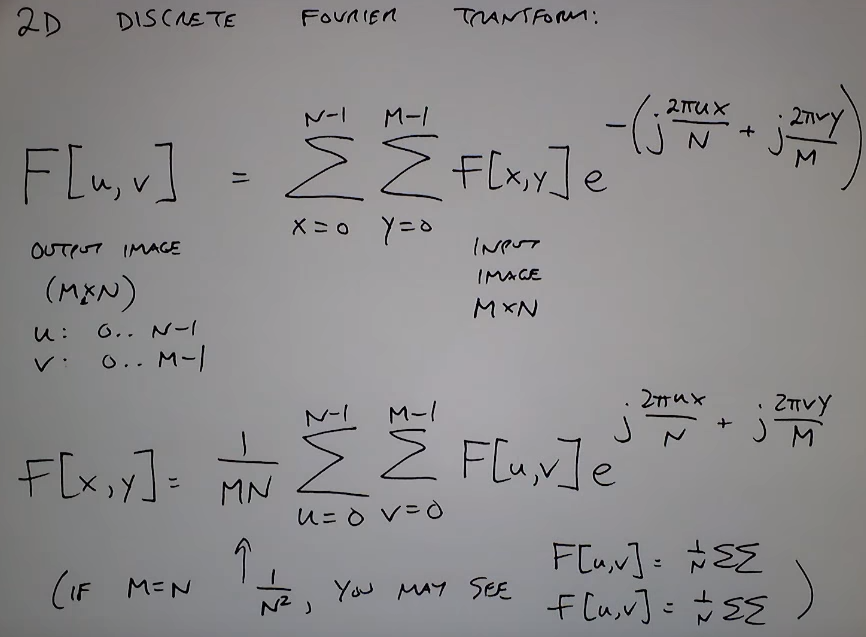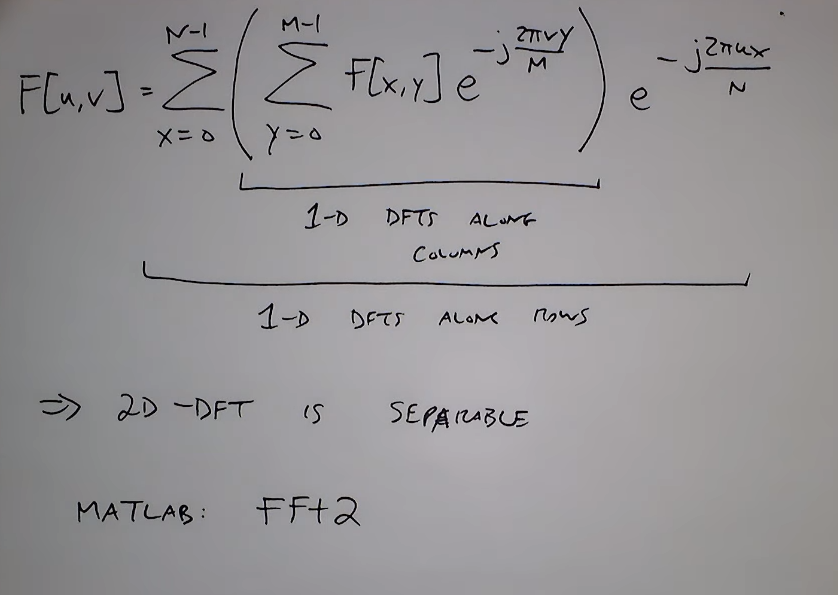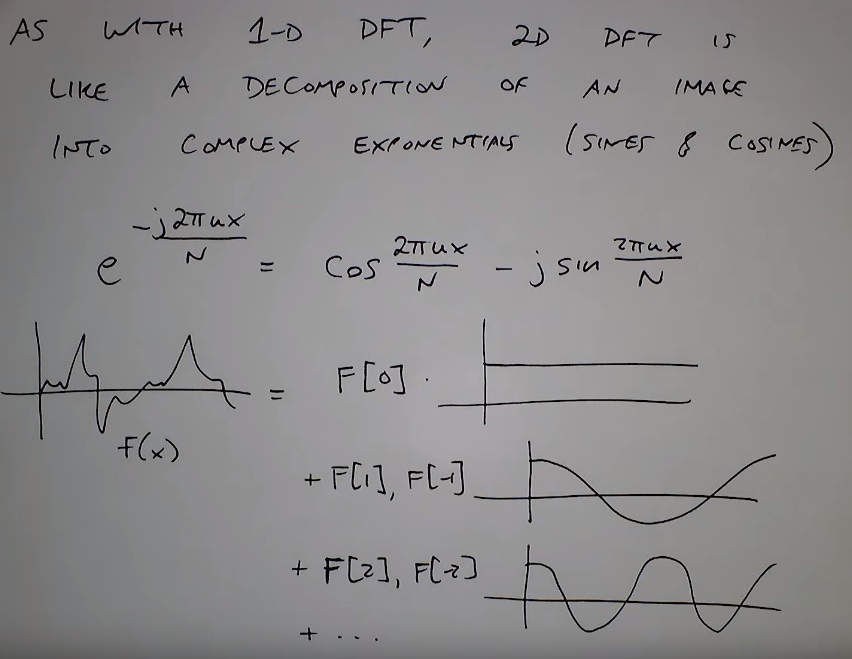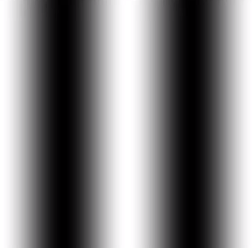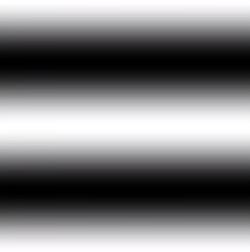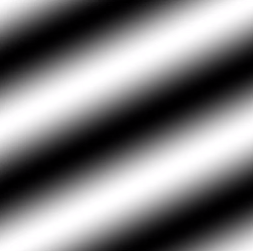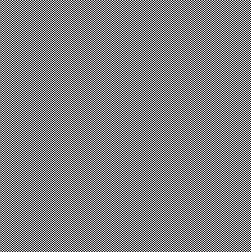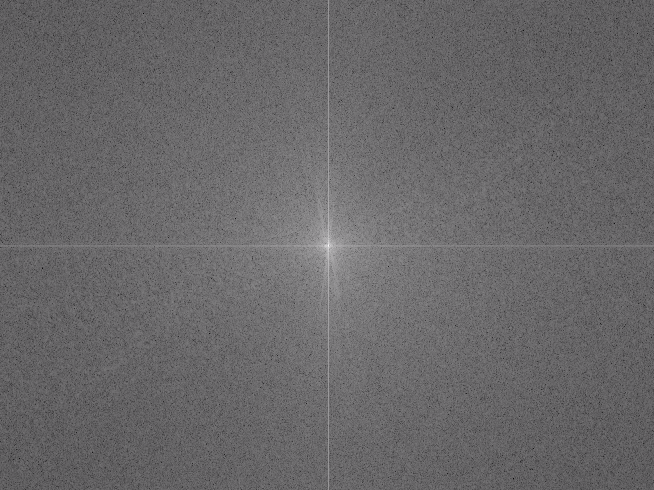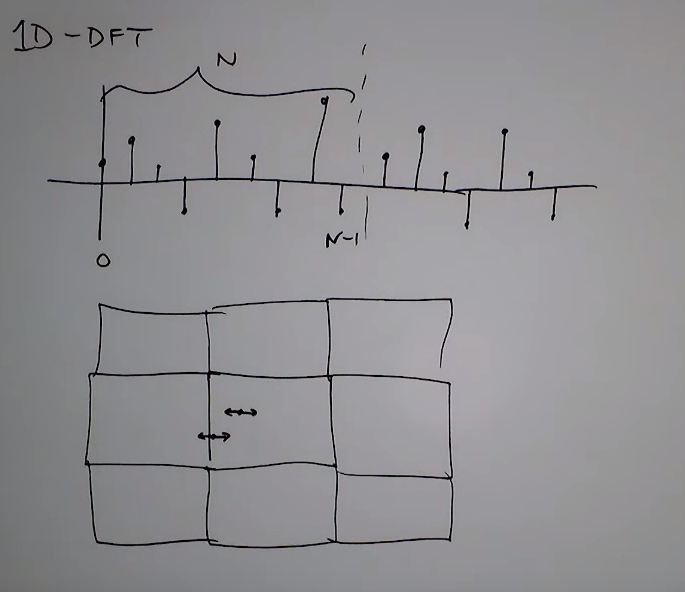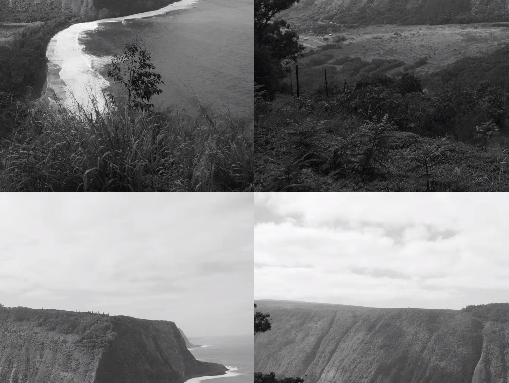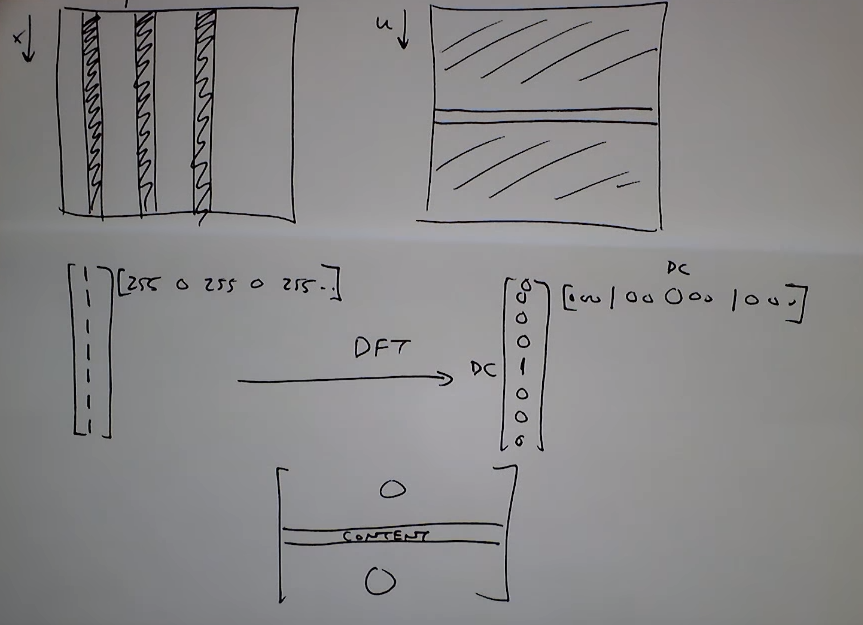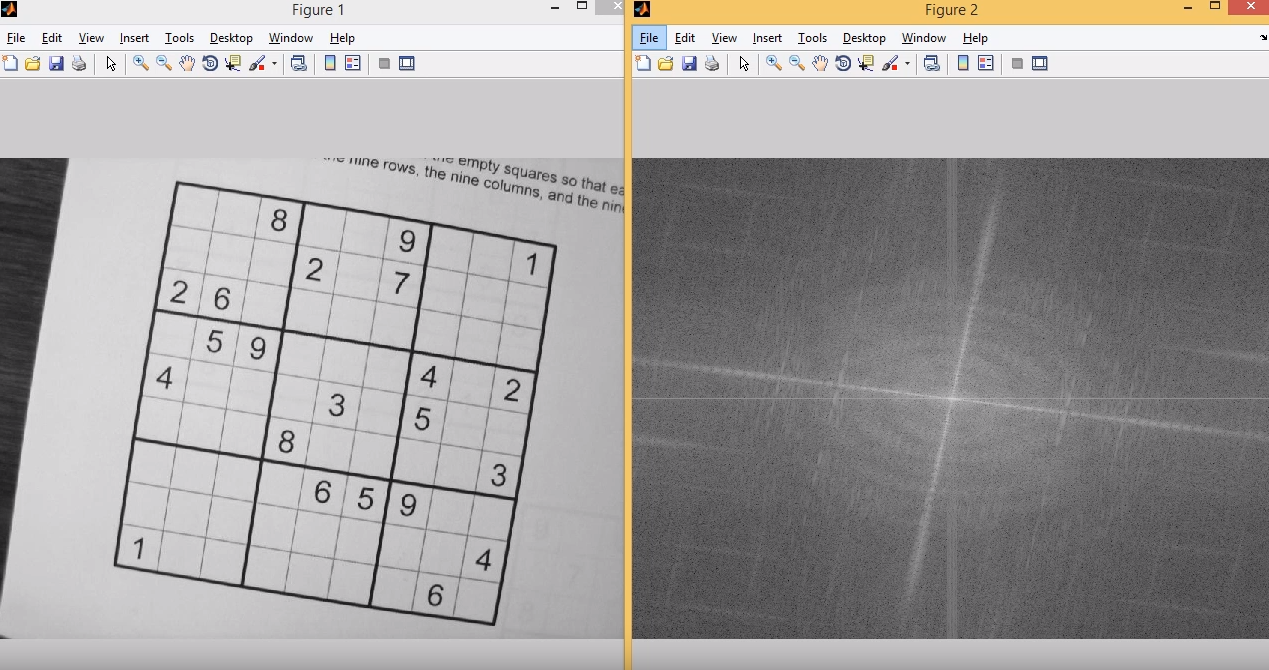# 二维傅里叶变换的性质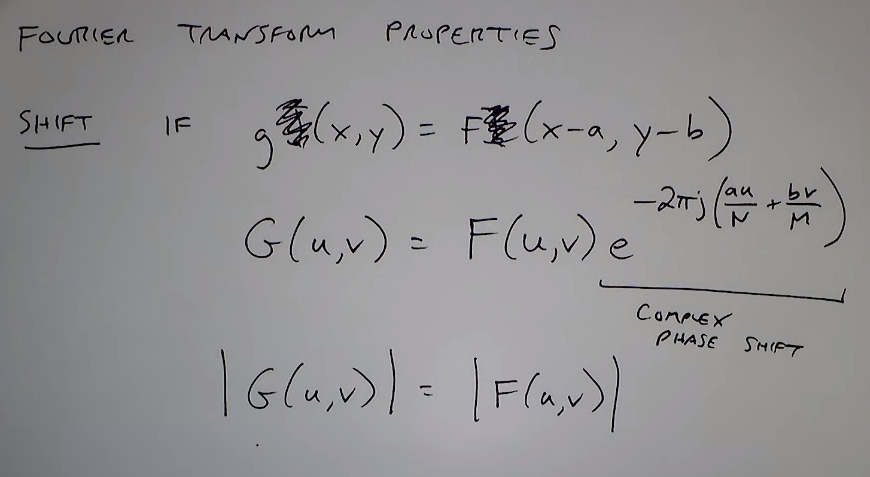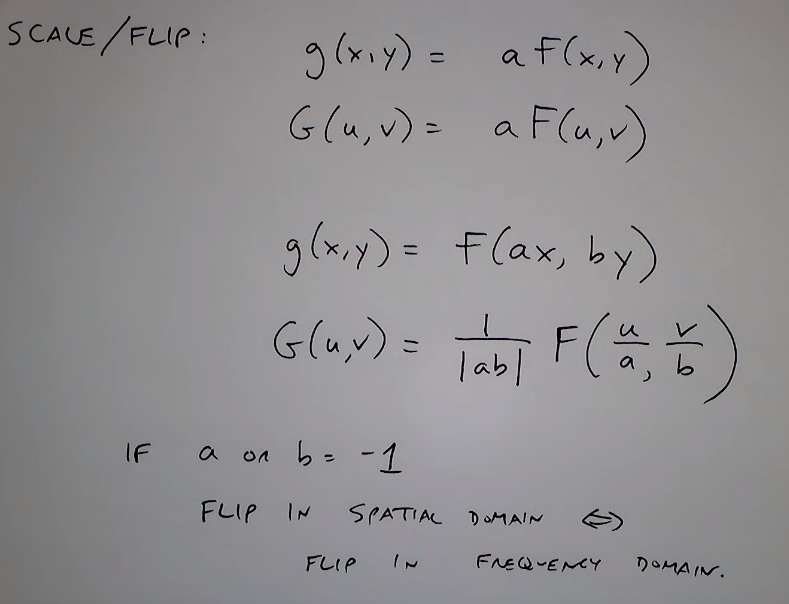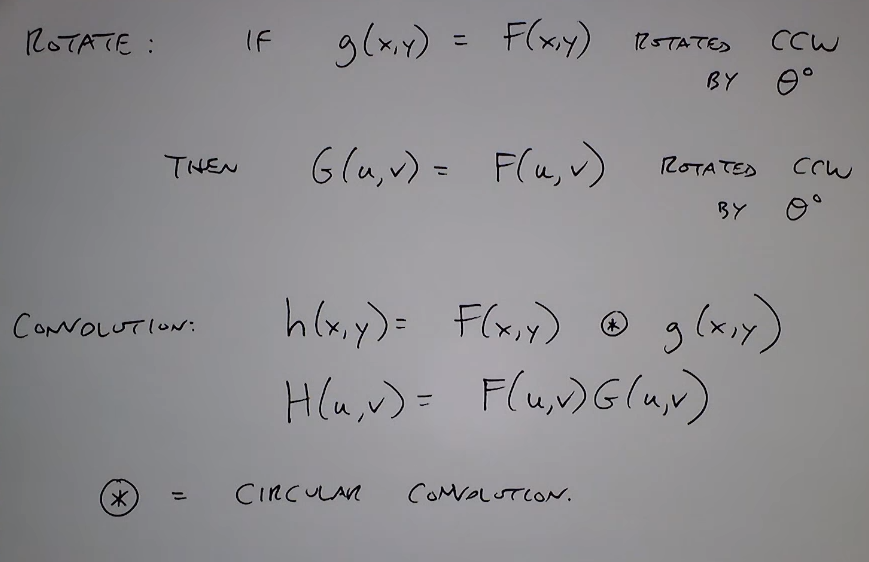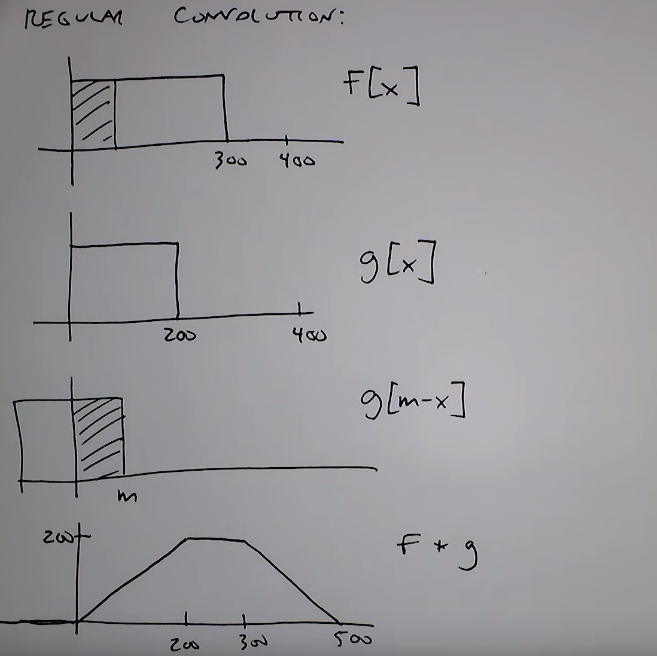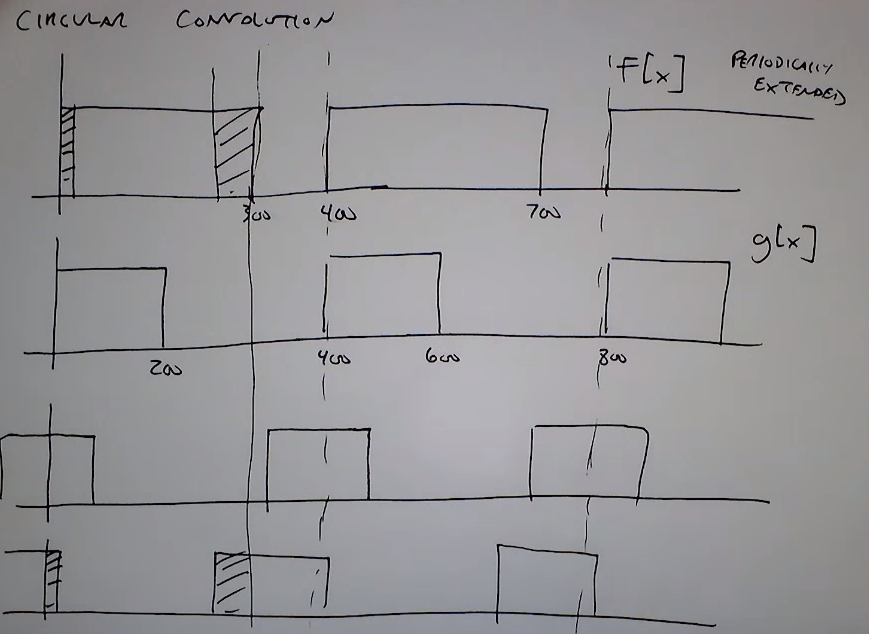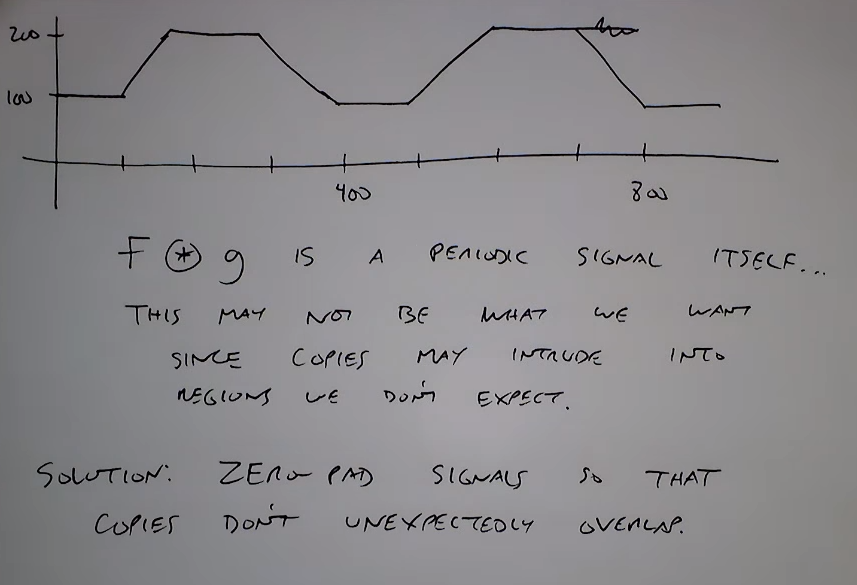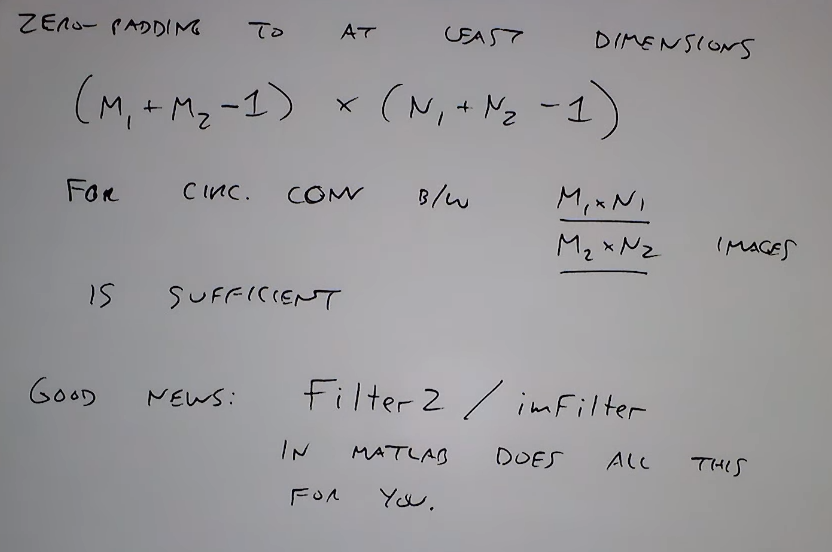2016-04-07 11:50:02 luokh327 阅读数 4554
• ###### 携手STM32CubeMX玩转STM32

754 人正在学习 去看看 李凯龙

1、简介

2、二维FFT、IFFT

对二维傅里叶变换及其逆变换的原理，可以查阅资料获得。这里给出其C语言实现。

在DSP中做计算时，涉及到很多内存的动态分配，这里采用的是固定内存分配的方式，即将内存直接使用#pragma        DATA_SECTION(IMG,"DDR2_VIDEO"); 的方式直接映射到SDRAM空间中。执行此操作还需要配置相应的内存连接文件.cmd

-c
-stack          0x00020000      /* Stack Size */
-heap           0x00020000      /* Heap Size */

MEMORY
{
VECS:       o = 0x00000000  l = 0x00000080
IRAM:       o = 0x00000080  l = 0x00007f80  /*  32 kBytes */
DRAM:       o = 0x00010000  l = 0x00008000  /*  32 kBytes */
DDR2:       o = 0x80000000  l = 0x04000000  /* 128 MBytes */
DDR2_VIDEO: o = 0x84000000  l = 0x04000000  /* 128 MBytes */
}

SECTIONS
{

.bss        >   DDR2
.cinit      >   DDR2
.cio        >   DDR2
.const      >   DDR2
.data       >   DDR2
.far        >   DDR2
.stack      >   DDR2
.switch     >   DDR2
.sysmem     >   DDR2
.text       >   DDR2
.ddr2       >   DDR2 
	.IMG     >  DDR2_VIDEO
.Source  >  DDR2_VIDEO
.Scale   > DDR2_VIDEO
.Out     >  DDR2_VIDEO
.tdd     > DDR2_VIDEO
.fdd     > DDR2_VIDEO
.W       > DDR2_VIDEO
.X1      >DDR2_VIDEO
.X2      > DDR2_VIDEO
.TempT   > DDR2_VIDEO
.TempF   > DDR2_VIDEO
}


3、滤波

对2中的FFT2d变换的结果采用退化函数进行退化，然后在频率域下进行滤波操作，滤波完成后进行二维傅里叶逆变换IFFT2d(),便可以得到经过滤波的图像。

4、结果展示2019-07-03 14:00:02 weixin_42902413 阅读数 157
• ###### 携手STM32CubeMX玩转STM32

754 人正在学习 去看看 李凯龙

### 傅里叶变换

傅里叶变换经常被用来分析不同滤波器的频率特性。可以使用2D傅里叶变换（DFT）分析图像的频域特性，实现DFT的一个快速算法被称为快速傅里叶变换（FFT）。

## 1. Numpy中的傅里叶变换

函数np.fft.fft2()可以对信号进行频率转换，输出结果是一个复杂的数组。

• 第一个参数是输入图像，要求灰度图；
• 第二个参数是可选的，决定输出数组的大小；一般输出数组和输入数组一样大，但是如果输出图像比输入图像大，输入图像就需要在进行FFT前补0；如果小，输入图像会被切割。

### 1）将频率为0的部分（直流分量）从图像的左上角移动到图像中心

用np.fft.fftshift()实现，代码如下：

import cv2
import numpy as np
from matplotlib import pyplot as plt
f = np.fft.fft2(img)
fshift = np.fft.fftshift(f)
magnitude_spectrum = 20*np.log(np.abs(fshift))
plt.subplot(121), plt.imshow(img, cmap='gray')
plt.title('Input Image'), plt.xticks([]), plt.yticks([])
plt.subplot(122), plt.imshow(magnitude_spectrum, cmap='gray')
plt.title('Magnititude Spectrum'), plt.xticks([]), plt.yticks([])
plt.show()


结果图：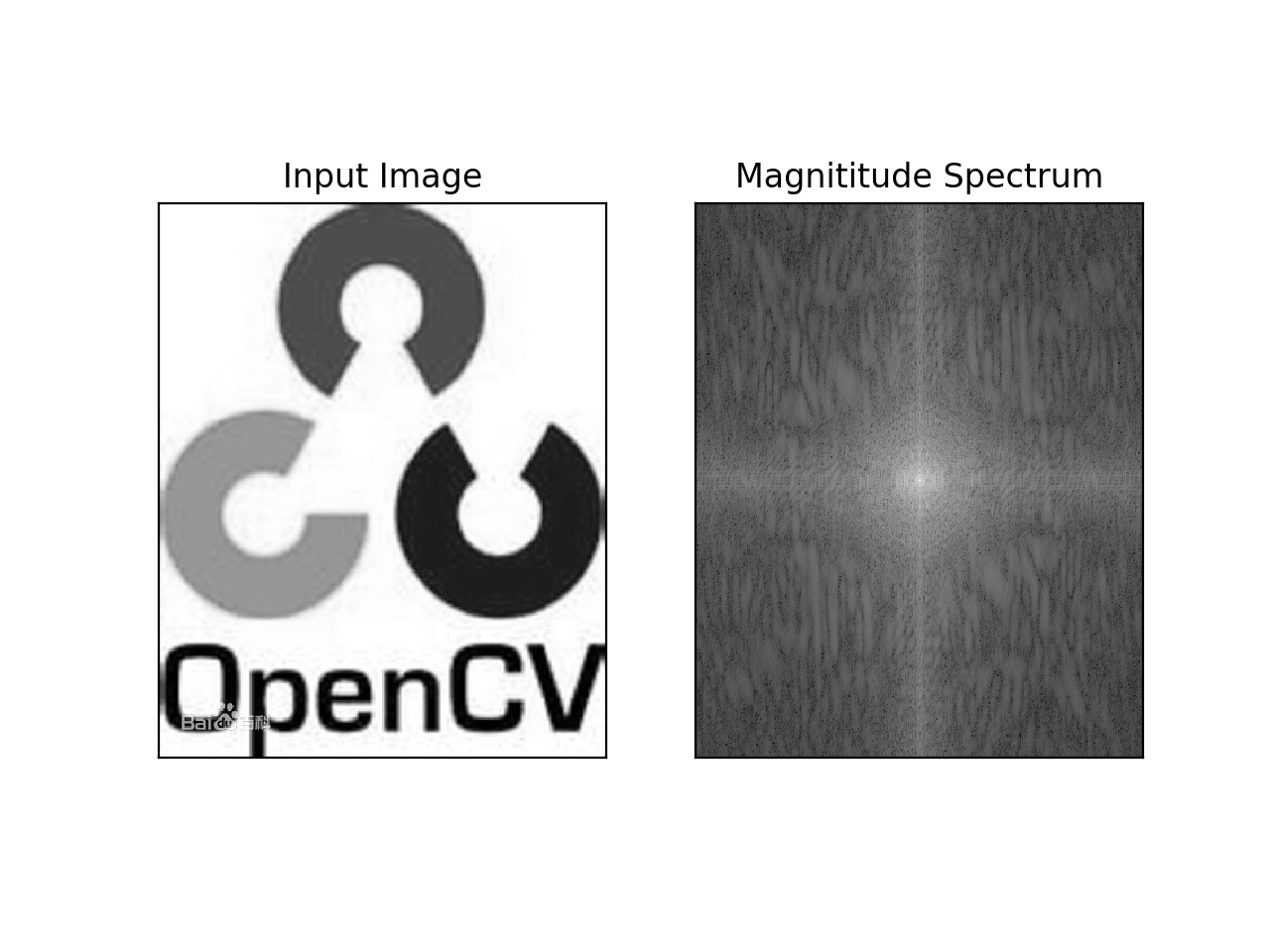### 2）频域变换

使用功能一个60*60的矩形窗口对图像进行掩模操作从而去除低频分量；然后在进行逆平移操作，最后进行FFT逆变换。代码如下：

rows, cols = img.shape
crow, ccol = rows//2, cols//2
fshift[crow-30:crow+30, ccol-30:ccol+30] = 0
f_ishift = np.fft.ifftshift(fshift)
img_back = np.fft.ifft2(f_ishift)
#取绝对值
img_back = np.abs(img_back)
plt.subplot(131), plt.imshow(img, cmap='gray')
plt.title('Input Image'), plt.xticks([]), plt.yticks([])
plt.subplot(132), plt.imshow(img_back, cmap='gray')
plt.title('Image after HPF'), plt.xticks([]), plt.yticks([])
plt.subplot(133), plt.imshow(img_back)
plt.title('Result in IET'), plt.xticks([]), plt.yticks([])
plt.show()


结果图：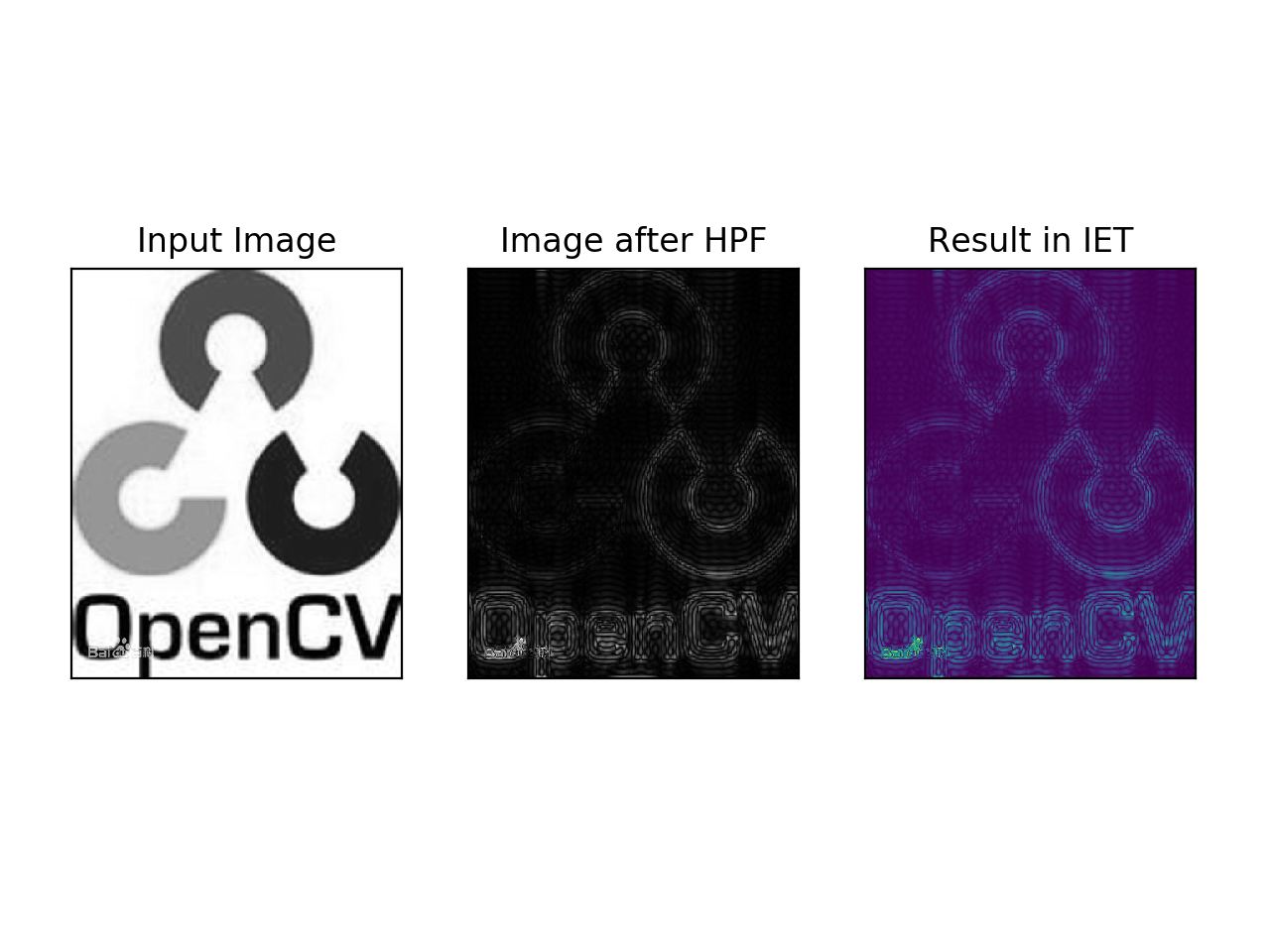从结果图看，高通滤波其实是一种边界检测操作。且由于用矩形窗口滤波出现了振铃效应，所以实际应用中，最好用高斯窗口进行滤波。

## 2. OpenCV中的傅里叶变换

### 1)直流分量平移

dft = cv2.dft(np.float32(img), flags=cv2.DFT_COMPLEX_OUTPUT)
dft_shift = np.fft.fftshift(dft)
magnitude_spectrum = 20*np.log(cv2.magnitude(dft_shift[:, :, 0], dft_shift[:, :, 1]))
plt.subplot(121), plt.imshow(img, cmap='gray')
plt.title('Input Image'), plt.xticks([]), plt.yticks([])
plt.subplot(122), plt.imshow(magnitude_spectrum, cmap='gray')
plt.title('magnitude spectrum'), plt.xticks([]), plt.yticks([])
plt.show()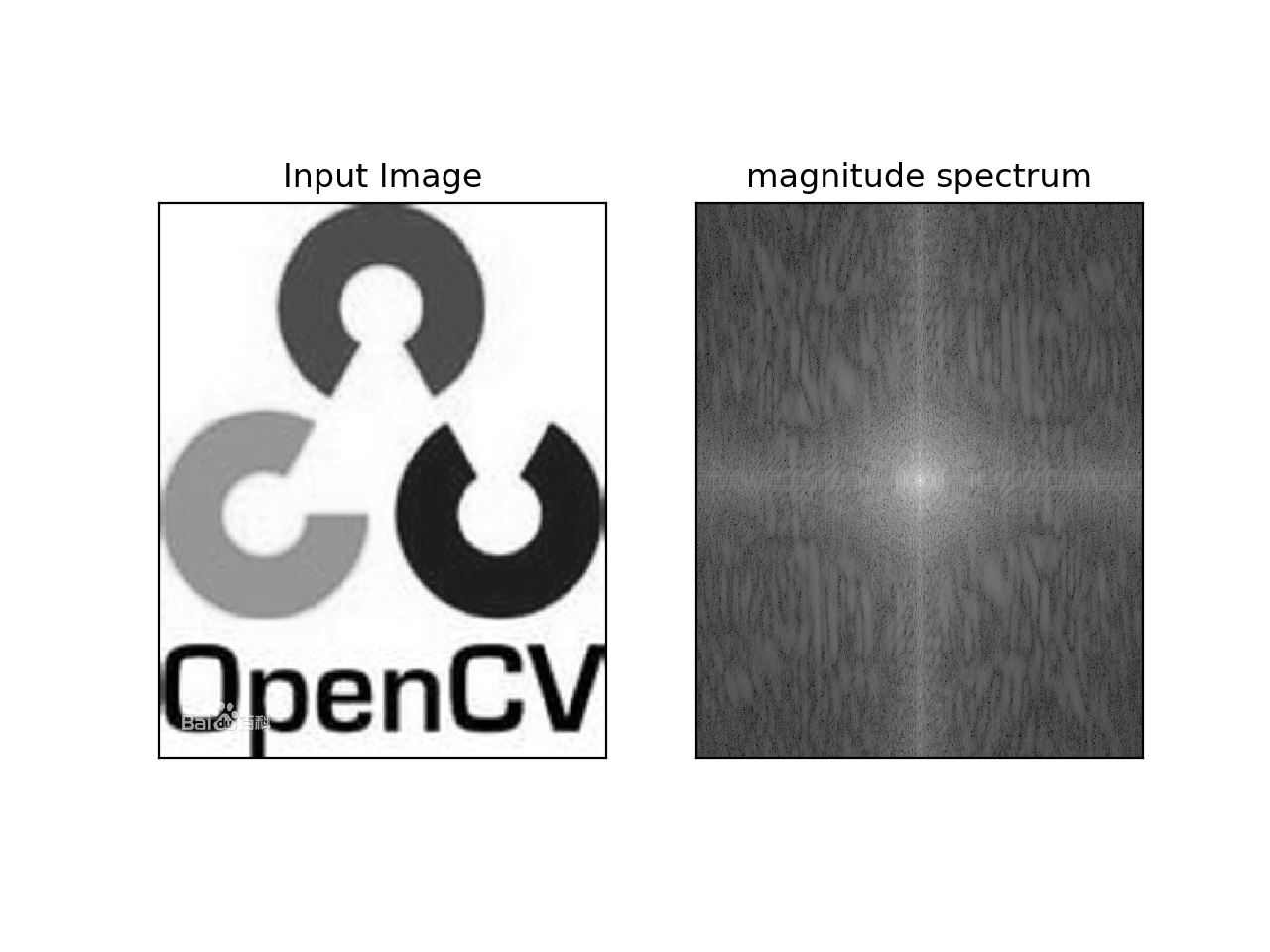### 2）逆DFT

• 先实现HPF（高通滤波）
• 再做LPF（低通滤波）将高频部分去除
rows, cols = img.shape
crow, ccol = rows//2, cols//2
#创建掩模，中心部分是1，其余为0
mask = np.zeros((rows, cols, 2), np.uint8)
# 逆DFT
f_ishift = np.fft.ifftshift(fshift)
img_back = cv2.idft(f_ishift)
img_back = cv2.magnitude(img_back[:, :, 0], img_back[:, :, 1])
plt.subplot(121), plt.imshow(img, cmap='gray')
plt.title('Input Image'), plt.xticks([]), plt.yticks([])
plt.subplot(122), plt.imshow(img_back, cmap='gray')
plt.title('img back'), plt.xticks([]), plt.yticks([])
plt.show()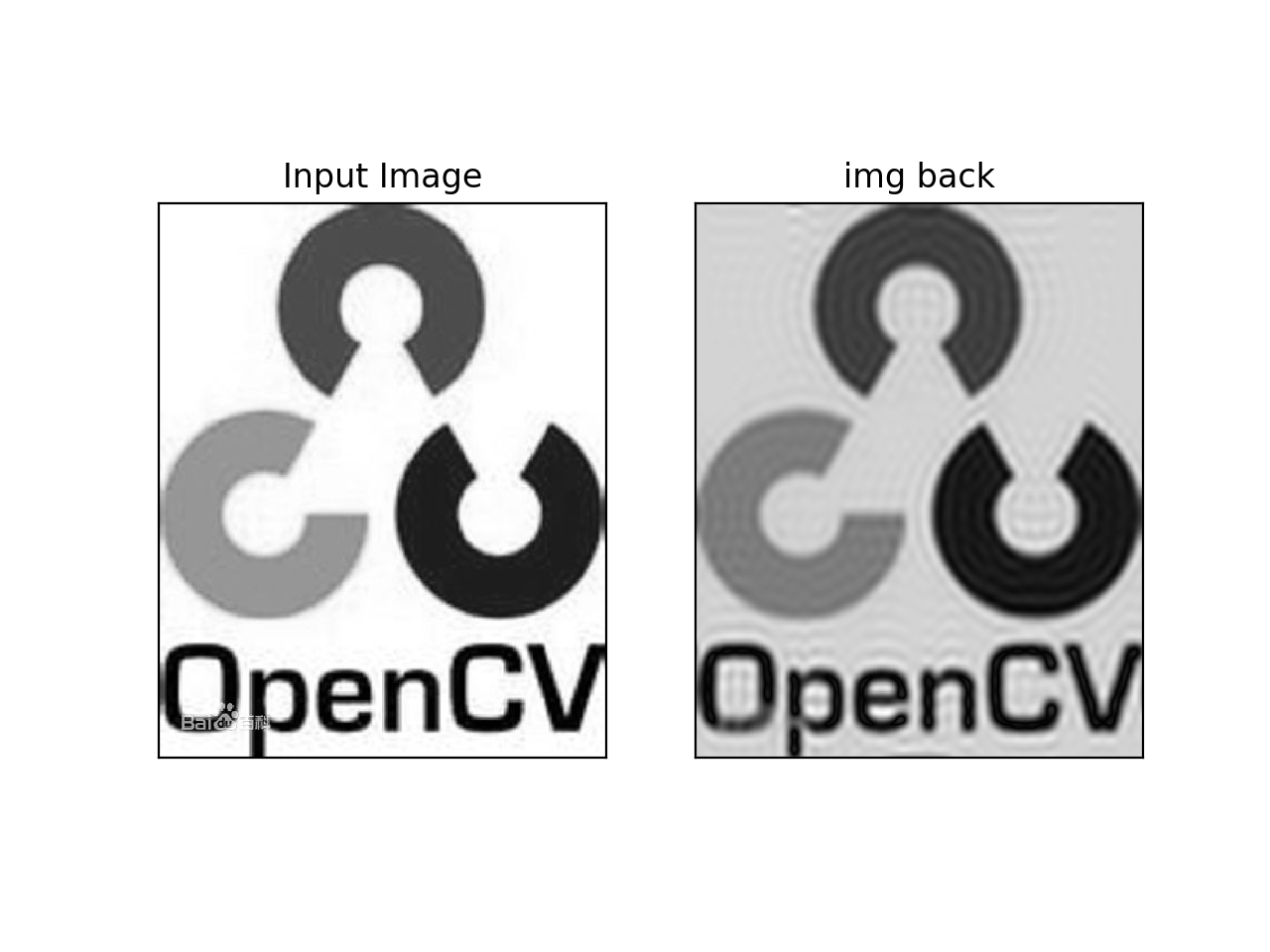cv2.dft() 和cv2.idft()要比Numpy快，但是Numpy更加用户友好。

图像处理2d傅立叶变换。 相关内容

2019-08-16 15:28:14 weixin_42555985 阅读数 50
• ###### 携手STM32CubeMX玩转STM32

754 人正在学习 去看看 李凯龙

官网 https://docs.opencv.org/3.4.1/de/dbc/tutorial_py_fourier_transform.html

傅里叶变换通常被用来分析各种滤波器的频率特性。
对于图像，2D离散傅里叶变换(DFT)被用于发现频域（frequency domain）。有一个计算DFT的快速算法叫快速傅里叶变换(FFT)。以上内容细节可以在任何一本图像处理或者信号处理的书中找到。

对于正弦信号
$x(t)=A sin(2πft)$
f是信号频率，如果把这个信号转到它的频域表示，我们可以在f中看到一个峰值。
如果对信号以离散形式采样，我们可以得到同样的频域（只不过是离散的），但是周期是 [−π,π] 或者 [0,2π] （N点DFT是 [0,N] ）。
你可以把图像看成是沿着2个方向采样的信号。所以对图像同时进行X方向和Y方向的傅里叶变换，就可以得到图像的频率表示（频谱图）。

更直观一点说，对于正弦信号，如果它的幅度在短时间内变化很快，你可以说它是高频信号。如果变化很慢，那就是低频信号。你可以把同样的想法应用于图像。

那么图像中的振幅在哪里变化剧烈呢？在边界点或者噪音。
所以我们可以说边界和噪音是图像的高频部分。图像中振幅变化不大的部分，就是低频部分。

## 1.numpy中的傅里叶变换

我们先来看看numpy中如何实现傅里叶变换。
numpy中有FFT包实现傅里叶变换。我们可以使用np.fft.fft2()函数进行频率变换，输出是一个复杂数组。
函数的第1个参数是输入的灰度图。第2个参数是可选的，输出数组的大小。如果输出数组比输入图像大，输入图像需要在计算FFT之前补0；如果比输入图像小，那么输入图像会被裁剪；默认输出和输入大小一样。

输出结果中，0频部分（直流部分）在左上角。如果你想把它移到图像中间，就需要沿2个方向平移 $\frac N2$。np.fft.fftshift()函数可以实现平移。

一旦完成频率变换后，就可以得到振幅谱。

例，获得图像振幅谱

import cv2
import numpy as np
from matplotlib import pyplot as plt

f = np.fft.fft2(img)
fshift = np.fft.fftshift(f)
magnitude_spectrum = 20*np.log(np.abs(fshift))

plt.subplot(121),plt.imshow(img, cmap = 'gray')
plt.title('Input Image'), plt.xticks([]), plt.yticks([])
plt.subplot(122),plt.imshow(magnitude_spectrum, cmap = 'gray')
plt.title('Magnitude Spectrum'),
plt.xticks([]), plt.yticks([])
plt.show()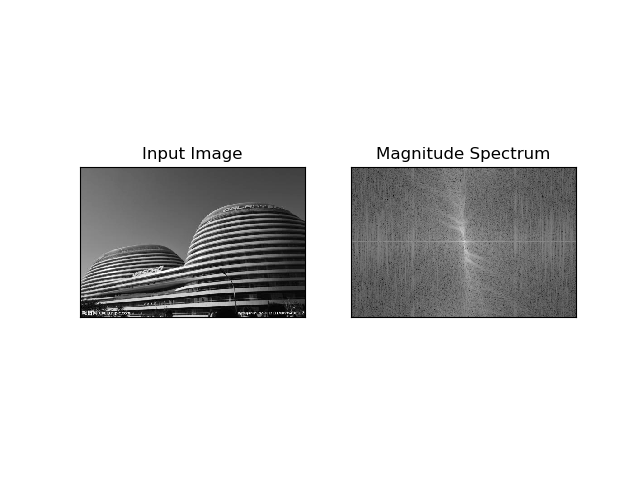结果如上图。
可以发现输出结果的中心部分更白（亮），这说明图像中的低频部分更多。

接下来你可以在频域中做一些操作了，比如高通滤波和图像重建（DFT的逆变换）。
你可以用一个60*60的矩形窗口做掩膜来去除低频部分。然后用np.fft.ifftshift() 函数进行逆平移操作，这样直流部分又回到左上角。最后用np.ifft2() 函数进行FFT逆变换。结果依然是复杂的数字，你可以取绝对值。

例，高通滤波和图像重建

import cv2
import numpy as np
from matplotlib import pyplot as plt

f = np.fft.fft2(img)
fshift = np.fft.fftshift(f)
magnitude_spectrum = 20*np.log(np.abs(fshift))

rows, cols = img.shape
crow,ccol = rows/2 , cols/2
fshift[crow-30:crow+30, ccol-30:ccol+30] = 0 #掩膜，去除低频部分
f_ishift = np.fft.ifftshift(fshift) #逆平移
img_back = np.fft.ifft2(f_ishift) #FFT逆变换
img_back = np.abs(img_back) #取绝对值

plt.subplot(131),plt.imshow(img, cmap = 'gray')
plt.title('Input Image'), plt.xticks([]), plt.yticks([])
plt.subplot(132),plt.imshow(img_back, cmap = 'gray')
plt.title('Image after HPF'), plt.xticks([]), plt.yticks([])
plt.subplot(133),plt.imshow(img_back)
plt.title('Result in JET'), plt.xticks([]), plt.yticks([])
plt.show()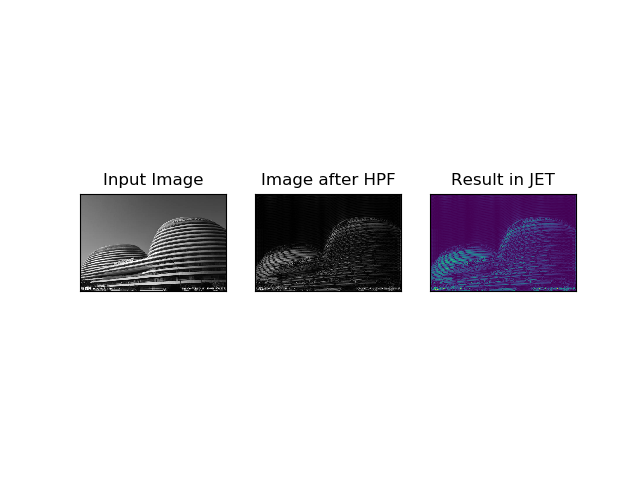结果如上图。
可以发现高通滤波实际是一种边界检测。
在《图像梯度》https://blog.csdn.net/weixin_42555985/article/details/96423427 中已经说过，高通滤波器主要是为了图像识别抽取出图像特征，这里的特征一般为边缘纹理的特征。因为图像中边缘和纹理细节都是高频信号。
同时，我们还可以发现图像中的大部分数据集中在频谱图的低频区域。

仔细观察最后一幅彩色JET图，你可以看到一些人工痕迹（把图放大可以看清）。在建筑物的上方有一些条带状的结构，这个被称为振铃效应（ringing effects）。这是因为我们使用矩形窗口做掩膜，掩膜被转换为正弦引起的。所以不要用矩形窗口作为过滤器，最好使用高斯窗口。

以上就是numpy中进行DFT和IDFT（逆）。

## 2.opencv中傅里叶变换

opencv提供cv.dft() 函数和cv.idft() 函数实现DFT和IDFT。它们会返回同样结果，但是是双通道的。第一个通道是结果的实数部分，第二个通道是结果的虚数部分。输入图像首先要转换为np.float32格式。

例，opencv实现DFT

import cv2
import numpy as np
from matplotlib import pyplot as plt

dft = cv2.dft(np.float32(img),flags = cv2.DFT_COMPLEX_OUTPUT)
dft_shift = np.fft.fftshift(dft)
magnitude_spectrum = 20*np.log(cv2.magnitude(dft_shift[:,:,0],dft_shift[:,:,1]))

plt.subplot(121),plt.imshow(img, cmap = 'gray')
plt.title('Input Image'), plt.xticks([]), plt.yticks([])
plt.subplot(122),plt.imshow(magnitude_spectrum, cmap = 'gray')
plt.title('Magnitude Spectrum'), plt.xticks([]), plt.yticks([])
plt.show()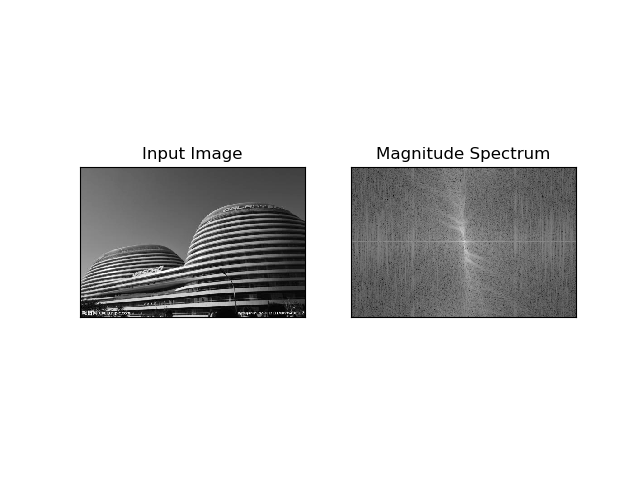结果如上图。

你可以使用cv.cartToPolar() 函数，它可以返回单个快照的振幅和相位。

下面来实现逆DFT。在上面numpy介绍中，我们创建了HPF（高通滤波），现在我们来看看如何去除图像的高频部分，也就是创建LPF（图像的低通滤波）。

为此，我们创建一个掩膜，低频部分都设置为1，高频部分设置为0，也就是让高频部分被过滤掉，低频部分保留。

例，创建LPF

import cv2
import numpy as np
from matplotlib import pyplot as plt

dft = cv2.dft(np.float32(img),flags = cv2.DFT_COMPLEX_OUTPUT)
dft_shift = np.fft.fftshift(dft)
# magnitude_spectrum = 20*np.log(cv2.magnitude(dft_shift[:,:,0],dft_shift[:,:,1]))

rows, cols = img.shape
crow,ccol = rows/2 , cols/2
# create a mask first, center square is 1, remaining all zeros
# apply mask and inverse DFT
f_ishift = np.fft.ifftshift(fshift)
img_back = cv2.idft(f_ishift)
img_back = cv2.magnitude(img_back[:,:,0],img_back[:,:,1])

plt.subplot(121),plt.imshow(img, cmap = 'gray')
plt.title('Input Image'), plt.xticks([]), plt.yticks([])
plt.subplot(122),plt.imshow(img_back, cmap = 'gray')
plt.title('Magnitude Spectrum'), plt.xticks([]), plt.yticks([])
plt.show()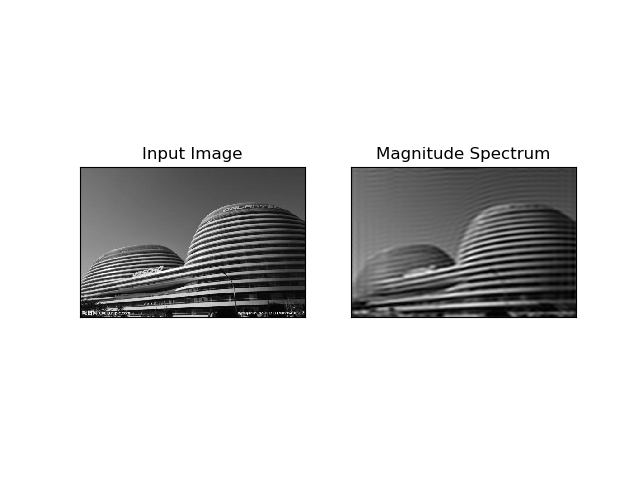结果如上图。

opencv中函数cv.dft()和cv.idft() 运算比numpy快。但是numpy函数更加友好。

## 3.DFT的性能优化

数组大小为某些值时候，DFT计算时性能更好。当数组大小是2的幂时候，DFT最快。当数组的大小是2，3，5 的倍数时效率也会很高。如果你担心代码的性能，可以在DFT之前修改输入图像的尺寸（填0）。opencv中必须手工填0。对于numpy，你只需要指定FFT计算的大小，它就可以自动填0。

如何找到最佳大小呢？opencv提供 cv.getOptimalDFTSize()函数。cv.dft() 和np.fft.fft2()函数都可以使用它。

import cv2
import numpy as np
import time
from matplotlib import pyplot as plt

rows,cols = img.shape
print "{} {}".format(rows,cols) #原尺寸 333 500

nrows = cv2.getOptimalDFTSize(rows)
ncols = cv2.getOptimalDFTSize(cols)
print "{} {}".format(nrows,ncols) #优化后 360 500

nimg = np.zeros((nrows,ncols)) #填0
nimg[:rows,:cols] = img


图像处理2d傅立叶变换。 相关内容

2019-05-04 20:27:54 cool__girls 阅读数 1294
• ###### 携手STM32CubeMX玩转STM32

754 人正在学习 去看看 李凯龙

打开任意图像，进行傅里叶变换，画出频谱图、相位谱图以及实现位移后的频谱图和相位谱图。
I=rgb2gray(I);
subplot(3,2,1);
imshow(I);
title(‘原始图像’);
F=fft2(I);
S=abs(F);
subplot(3,2,2);
imshow(S,[]);
title(‘频谱图’);
Fc=fftshift(F);
subplot(3,2,3);
imshow(abs(Fc),[]);
title(‘移频后’);
s2=log(1+abs(Fc));
subplot(3,2,4);
imshow(s2,[]);
title(‘log增强后的频谱图’);
r=real(F);
i=imag(F);
s1=log(1+abs(F));
phase=angle(F)*180/pi;
I=rgb2gray(I);
subplot(3,2,1);
imshow(I);
title(‘原始图像’);
F=fft2(I);
S=abs(F);
subplot(3,2,2);
imshow(S,[]);
title(‘频谱图’);
Fc=fftshift(F);
subplot(3,2,3);
imshow(abs(Fc),[]);
title(‘移频后’);
s2=log(1+abs(Fc));
subplot(3,2,4);
imshow(s2,[]);
title(‘log增强后的频谱图’);
r=real(F);
i=imag(F);
s1=log(1+abs(F));
phase=angle(F)*180/pi;
subplot(3,2,5);
imshow(phase,[]);
title(‘相位谱图’);
r1=real(Fc);
i1=imag(Fc);
phase2=angle(Fc)*180/pi;
subplot(3,2,6);
imshow(phase2);
title(‘位移后相位谱图’);
imshow(phase,[]);
title(‘相位谱图’);
r1=real(Fc);
i1=imag(Fc);
phase2=angle(Fc)*180/pi;
subplot(3,2,6);
imshow(phase2);
title(‘位移后相位谱图’);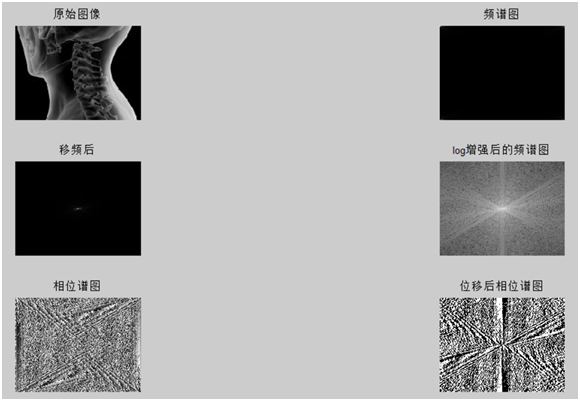# 欢迎使用Markdown编辑器

你好！ 这是你第一次使用 Markdown编辑器 所展示的欢迎页。如果你想学习如何使用Markdown编辑器, 可以仔细阅读这篇文章，了解一下Markdown的基本语法知识。

## 新的改变

我们对Markdown编辑器进行了一些功能拓展与语法支持，除了标准的Markdown编辑器功能，我们增加了如下几点新功能，帮助你用它写博客：

1. 全新的界面设计 ，将会带来全新的写作体验；
2. 在创作中心设置你喜爱的代码高亮样式，Markdown 将代码片显示选择的高亮样式 进行展示；
3. 增加了 图片拖拽 功能，你可以将本地的图片直接拖拽到编辑区域直接展示；
4. 全新的 KaTeX数学公式 语法；
5. 增加了支持甘特图的mermaid语法1 功能；
6. 增加了 多屏幕编辑 Markdown文章功能；
7. 增加了 焦点写作模式、预览模式、简洁写作模式、左右区域同步滚轮设置 等功能，功能按钮位于编辑区域与预览区域中间；
8. 增加了 检查列表 功能。

## 功能快捷键

撤销：Ctrl/Command + Z
重做：Ctrl/Command + Y
加粗：Ctrl/Command + B
斜体：Ctrl/Command + I
标题：Ctrl/Command + Shift + H
无序列表：Ctrl/Command + Shift + U
有序列表：Ctrl/Command + Shift + O
检查列表：Ctrl/Command + Shift + C
插入代码：Ctrl/Command + Shift + K
插入链接：Ctrl/Command + Shift + L
插入图片：Ctrl/Command + Shift + G

## 合理的创建标题，有助于目录的生成

直接输入1次#，并按下space后，将生成1级标题。
输入2次#，并按下space后，将生成2级标题。
以此类推，我们支持6级标题。有助于使用TOC语法后生成一个完美的目录。

## 如何改变文本的样式

强调文本 强调文本

加粗文本 加粗文本

标记文本

删除文本

引用文本

H2O is是液体。

210 运算结果是 1024.

## 插入链接与图片

图片:带尺寸的图片:居中的图片:居中并且带尺寸的图片:当然，我们为了让用户更加便捷，我们增加了图片拖拽功能。

## 如何插入一段漂亮的代码片

博客设置页面，选择一款你喜欢的代码片高亮样式，下面展示同样高亮的 代码片.

// An highlighted block
var foo = 'bar';


• 项目
• 项目
• 项目
1. 项目1
2. 项目2
3. 项目3
• 计划任务
• 完成任务

## 创建一个表格

一个简单的表格是这么创建的：

项目 Value
电脑 $1600 手机$12
导管 \$1

### 设定内容居中、居左、居右

使用:---------:居中
使用:----------居左
使用----------:居右

第一列 第二列 第三列
第一列文本居中 第二列文本居右 第三列文本居左

### SmartyPants

SmartyPants将ASCII标点字符转换为“智能”印刷标点HTML实体。例如：

TYPE ASCII HTML
Single backticks 'Isn't this fun?' ‘Isn’t this fun?’
Quotes "Isn't this fun?" “Isn’t this fun?”
Dashes -- is en-dash, --- is em-dash – is en-dash, — is em-dash

## 创建一个自定义列表

Markdown
Text-to-HTML conversion tool
Authors
John
Luke

一个具有注脚的文本。2

## 注释也是必不可少的

Markdown将文本转换为 HTML

## KaTeX数学公式

您可以使用渲染LaTeX数学表达式 KaTeX:

Gamma公式展示 $\Gamma(n) = (n-1)!\quad\forall n\in\mathbb N$ 是通过欧拉积分

$\Gamma(z) = \int_0^\infty t^{z-1}e^{-t}dt\,.$

你可以找到更多关于的信息 LaTeX 数学表达式here.

## 新的甘特图功能，丰富你的文章

• 关于 甘特图 语法，参考 这儿,

## UML 图表

可以使用UML图表进行渲染。 Mermaid. 例如下面产生的一个序列图：:

这将产生一个流程图。:

• 关于 Mermaid 语法，参考 这儿,

## FLowchart流程图

我们依旧会支持flowchart的流程图：

• 关于 Flowchart流程图 语法，参考 这儿.

## 导出与导入

### 导出

如果你想尝试使用此编辑器, 你可以在此篇文章任意编辑。当你完成了一篇文章的写作, 在上方工具栏找到 文章导出 ，生成一个.md文件或者.html文件进行本地保存。

### 导入

如果你想加载一篇你写过的.md文件或者.html文件，在上方工具栏可以选择导入功能进行对应扩展名的文件导入，
继续你的创作。

1. 注脚的解释 ↩︎

图像处理2d傅立叶变换。 相关内容

阅读数 2693

博文 来自： ylj135cool

阅读数 538

博文 来自： l1987021

阅读数 518

博文 来自： yukinoai
博文 来自： qq_41905045

阅读数 289

博文 来自： tianyu_lei
没有更多推荐了，返回首页